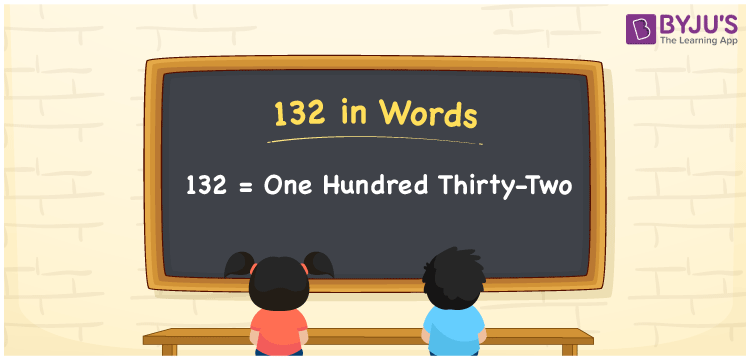# 132 in Words

We can write 132 in words as One Hundred Thirty-Two. For instance, the cost of a school bag is Rs. 132, then you can say, “The cost of a school bag is Rupees One Hundred Thirty-Two”. We know that 132 is a cardinal number as it depicts a specific amount. The place value chart helps a lot to convert any number into words quickly. Let us understand how to represent the number name for 132 using a place value table in this article.

 132 in Words One Hundred Thirty-Two One Hundred Thirty-Two in numerical form 132

## 132 in English Words

We generally represent the numbers in words using the letters of the English alphabet. Hence, the number 132 in English is read as One Hundred Thirty-Two.## How to Write 132 in Words?

The place value chart for the number 132 is as follows.

 Hundreds Tens Ones 1 3 2

Therefore, we can write the expanded form as:

1 x Hundred + 3 x Ten + 2 x One

= 1 x 100 + 3 x 10 + 2 x 1

= 100 + 30 + 2

= 132

= One Hundred Thirty-Two

Hence, 132 in words is written as One Hundred Thirty-Two

Interesting way of writing 132 in words

1 = One

13 = Thirteen

132 = One Hundred Thirty-Two

Thus, the word form of the number 132 is One Hundred Thirty-Two

132 is a natural number that is one less than 133 and one greater than 131

• 132 in words – One Hundred Thirty-Two
• Is 132 an odd number? – No
• Is 132 an even number? – Yes
• Is 132 a perfect square number? – No
• Is 132 a perfect cube number? – No
• Is 132 a prime number? – No
• Is 132 a composite number? – Yes

## Frequently Asked Questions on 132 in Words

### How do you write 132 in words?

132 in words is written as One Hundred Thirty-Two.

### What is the place value of 3 in 132?

The place value of 3 in 132 is Tens.

### 132 is an odd number. True or False.

False, the number 132 is not an odd number.